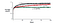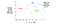# Introductory notes on Reinforcement learning

## Overview

This post covers introductory aspects of reinforcement learning. There has been a lot of buzz around reinforcement learning lately with many new applications getting mainstream visibility. One such example is a where a reinforcement learning based system AlphaGO has defeated many prominent GO world champions. Another cool example of reinforcement learning came from DeepMind, where they were able to train a model to play some games on Atari. In addition, many systems problems might be solvable using reinforcement learning techniques. This post covers some of the introductory aspects of reinforcement learning mostly chapter 1 and some aspects of chapter 2 from this book.

## What is Reinforcement learning?

In a nutshell, reinforcement learning consists of an agent interacting with an environment and reacting to the sensory input from the environment by taking some actions. These actions get rewarded so as to direct the agent to make more optimal decisions going forward. The agent is not told what actions to take; but must discover those by trial and error. In addition, reward doesn’t only mean the immediate reward, but the reward in the long run. These two aspects: trial-and-error search and delayed award form the most fundamental aspects of reinforcement learning.

Trial-and-error search mentioned above can also be thought of as explore and exploit dilemma. An agent interacting with the environment, needs to take actions that have been useful before — this is exploitation of known facts. But agent cannot know which actions lead to better results without exploring different actions which may not be optimal in the short term.

Delayed Reward is where a lot of research in reinforcement learning is focused on. There is an immediate reward and a long term goal. Greedy approaches may not always lead to most optimized results. How to define the long term value function for any action becomes a critical aspect of reinforcement learning. Many learning methods in that sense focus on estimating the value functions.

## How is reinforcement learning different from supervised and unsupervised learning?

In supervised learning, a training set labeled by a knowledgeable expert instructs the system about expected results — whether a given picture contains cat or not. The model is then supposed to generalize from that labeled data to figure out if a previously unseen picture does indeed contain a cat or not. In reinforcement learning, an agent is supposed to learn from its own action, there are no labeled samples. Agent that is interacting with environment may not have any correct answer or it might be impractical to label a correct answer for all the different scenarios.

One then might be tempted to think of this as an unsupervised learning problem. In unsupervised learning, the system tries to uncover the patterns hidden within the data. This could be useful in reinforcement learning, but is not sufficient. Agent needs to maximize the reward signal irrespective of finding patterns in data.

## Major components of a reinforcement learning system

There are 4 major components of any reinforcement learning system with the fourth one(environment model) being optional.

Policy: Policy is a mapping that represents what actions to take in the current state of the environment. In simple cases, given the current state of the environment, this can be a lookup table that can be used to take the next action by the agent.

Reward signal: Reward signal generally represents a numerical number that indicates to the agent whether the given action is positive or not. A low reward will indicate to the agent that this action yielded pain in the short-term. So, in future, the agent may select a different action to get better reward.

Value function: Value is a reflection on how much reward the agent is likely to accrue going forward from the given state. Does the current action in the current state yield to accumulation of more rewards over time? It is possible that the current action that has given the most reward is followed by lots of low reward states or an immediate low reward state is followed by many high reward states. In that sense, reward is immediate while value is delayed. Value is also not too useful without rewards — the only purpose of using the right value function is to accumulate more rewards in the long run. Estimating value is one of the most fundamental aspects of reinforcement learning.

Model of the environment: Model mimics the environment. Generally this is useful for predicting how the environment will behave given the current state and action — what would be the reward, given the current state and the action. Models can be useful in deciding course of actions without actually experiencing all the scenarios.

## A tabular method for Reinforcement learning

For simpler problems, it is possible to arrive at optimal solutions by keeping a lookup table of values that can be used for selecting actions. Let’s look into an example: A K-armed bandit problem. The problem is relatively simple. There are k options to select from and there is a reward associated with selecting any of those options. Over many steps, the idea is to maximize the value by selecting some actions during every step.

Let’s assume that we can maintain value estimates of each actions. Thus during every time step, we can select an action with the highest value. Selecting such action can be called a greedy action. But we would also like to explore and learn more other actions so as to improve their estimates going forward. These are the non-greedy actions. Having more uncertainty about non-greedy actions may imply that there are those actions which can lead to more value and hence greedy actions may not be the only choice. If we had known values for every action with certainty, then these exploration would not be needed. There are many complicated ways to balance this trade-off between exploration(non-greedy) and exploitation(greedy), but we will only stick to doing both somewhat here. Also subsequent results will show that non-greedy solutions will lead to more optimal results in long term value.

To solve this problem more concretely, we can use average-so-far as an estimate of the value of selecting the current action. This can be done by easily maintaining a running average for every action type and then updating the average each time an action is selected. In greedy mode, we would always select action type with max value, but since we want to explore as well, we select non-greedy action from all other actions with some small probability ε. Such methods are called as ε-greedy methods. An advantage of this methods is that, with many time steps, it ensures that all actions will be selected enough times to ensure almost certain value estimation. Experiments from the book(figure below) clearly show how ε-greedy with ε values of 0.1 and 0.01 outperform fully greedy options.As number of steps increase, more exploratory approach of ε = 0.1 leads to more average reward.

This method is called a tabular method because it can be implemented using a simple table that stores the running average so far for every action. A simple formula for running value average Q at n+1th time step can be expressed as:

Q(n+1) = Q(n) + 1/n * (R(n) — Q(n))

Here R(n) is the reward obtained at the nth step. Another way to think about this formula is: NewEstimate = OldEstimate + StepSize * (Target- OldEstimate).

In this case all the past rewards are weighted equally. This works well if the probability distributions and problem spaces are stable. If those were to change, then it could be more useful to give more weight to recent selections. This can be done altering the same formula to use a constant 0 < ⍺ ≤1.

Q(n+1) = Q(n) + ⍺ * ( R(n) — Q(n))

Biases

In above value-action scenarios, all averages were dependent on Q(1) i.e initial value, which is called as a bias into the algorithm. Generally for stationary non-alpha cases, the bias disappears once we select all actions. But for alpha based cases bias is permanent and only decreases with time.

One possible use of bias is to use an optimistic value for Q1. For example, in the K-arms bandit problem, if we choose a value that is off from the mean by quite a bit, then it will lead to more early exploration — this happens because Target — Q(1) will be wildly off and all actions will look inappropriate, forcing the algorithm to explore more. Generally speaking this is not a good strategy for sustainable exploration given that it is based on temporary initial condition.

## Selecting next actions using uncertainty metric(UCB)

In ε-greedy, we discussed that when we selected the next action for exploration it was picked with uniform probability from all the remaining non-greedy actions. It would be good to select the next action with some better heuristic. One such heuristic is called Upper-Confidence-Bound selection. In this measure, we select an action that maximizes:

Q(t) + c * ( sqrt(ln t/N(a))

What this formula tries to do is to add an uncertainty measure for the given action a. N(a) indicates the number of times a has been selected so far. If ‘a’ has never been selected then sqrt term is maximized and leads to selection of ‘a’. Otherwise as ‘a’ gets selected more and more times, the sqrt term becomes smaller — increasing the certainty. Similarly when any other action other than ‘a’ is selected, it increases the value of sqrt term, but slowly. This causes actions that have been often but with lower value, to not get selected after a while. It also causes to accrue more certainty around actions that haven’t been selected before.

## Gradient Bandit Algorithm

All the previous approaches mentioned below estimate values for every action. Another way could be to assign a priority/probability, at time t, H(t)(a) to every action and then select actions accordingly. Higher H(t)(a), more chances of it being selected. Once we have H(t)(a) — relative priorities, we can then use softmax to land relative probabilities(Pa) for all K actions.

Ideas of stochastic gradient descent to improve values of Ht(a) with every time step seem possible in this case. If R’ is the baseline reward so far, then we can use the following equation for improving Ht(a) with every step with ⍺ as the step size used in gradient descent.

H(t+1)(a) = H(t)(a) + ⍺ (R — R’)(1-Pa)

H(t+1)(a’) = H(t)(a’) — ⍺ (R — R’)(Pa’)

If reward R > R’, then positive feedback improves H(t+1)(a). At the same time, the second equation decreases the H(t+1)(a’) for all other actions a’. One can see the opposite effect when obtained reward R < baseline reward R’.

## Summary

We discussed basic aspects of reinforcement learning and some ways to manage the trade off between exploration and exploitation. All three methods ε-greedy, UCB, Gradient bandit achieve those trade offs by altering some parameter values.Reward values for different methods by varying related parameters

As it can be seen for k-bandits problem form U-graphs, all methods have an optimal value of the parameter where they perform the best. UCB seems to outperform other methods at least for this problem.

Welcome to a place where words matter. On Medium, smart voices and original ideas take center stage - with no ads in sight. Watch
Follow all the topics you care about, and we’ll deliver the best stories for you to your homepage and inbox. Explore
Get unlimited access to the best stories on Medium — and support writers while you’re at it. Just \$5/month. Upgrade